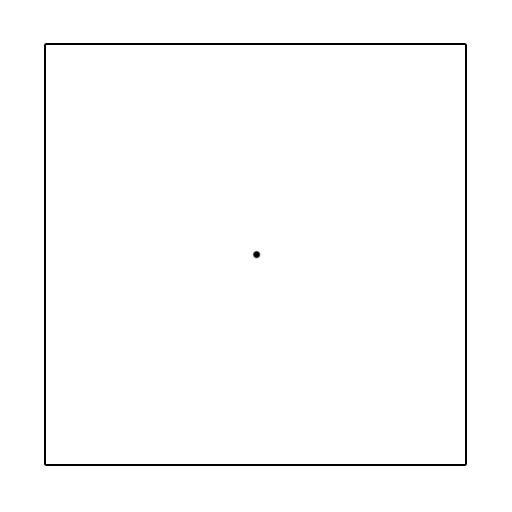# The Square Cross-over Part (a)

Probability Level 2Three points are chosen at random along the square's outline. These points are then combined to form a triangle (or a straight line if all 3 points are chosen on the same side). What is the probability that the center of the square will be contained within a triangle formed by these 3 points?

×